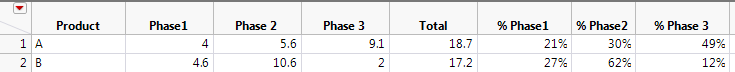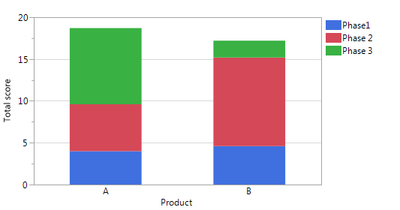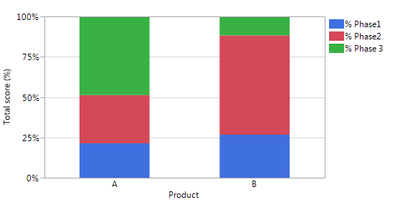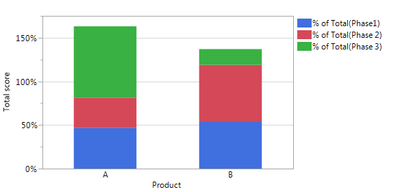Our World Statistics Day conversations have been a great reminder of how much statistics can inform our lives. Do you have an example of how statistics has made a difference in your life? Share your story with the Community!
Choose Language Hide Translation Bar
Highlighted

## Stacked bar - show % contribution of each column (using Graph builder)

Dear all,

I am struggling in making a graph using the graph builder for something that is probably fairly easy…

I have the following fictional dataset, with 2 products A and B and for each of them a score in 3 categories/phases. This can easily be shown with a stacked bar.FIG1:Now I want to see the results in % contributions to the total score each product has. I know I can do this by first summing the 3 columns Phase 1->3, and then divide the Phase1 score by this total etc, as I now manually did in the table. And then again, you can make a stacked bar to get the graph I need.

FIG2:So this definitely works. However, I now have a whole lot of columns to analyse, so manually dividing each column by the total seems like a big hurdle… Is there any fast way of converting FIG1 into FIG2??

I already tried with changing “summary statistic = % of total” in FIG1, but then my total is not 100% as shown in FIG3, so I think this gives me the % of total per column, while I want % of total per row (for those columns displayed in the chart).Yanne

1 ACCEPTED SOLUTION

Accepted Solutions
HighlightedjulianCommunity Manager

## Re: Stacked bar - show % contribution of each column (using Graph builder)

Hi @yanne,

As you have discovered, to make this plot in Graph Builder you will need to create the % of total formula columns. I have to do this sort of thing pretty often (calculate % of total columns for a set of columns in a data table) and to streamline the process I put together a simple Add-in to do it. I just uploaded that Add-in to the file exchange here:

I hope this helps!

Julian

3 REPLIES 3
Highlighted

## Re: Stacked bar - show % contribution of each column (using Graph builder)

Hello Yanne ,
You can probably try something like this , I proceed with a couple of assumptions here
Assumptions:
1. You capture the data table and its reference is "dt"

dt = Current Data Table();
ColNames = Get Column Names("String");
Mat = dt << Get As Matrix(); // Get the numeric data out of the data table
ColSum = VSum(Transpose(Mat));
dt << New Column("Totals",Numeric,Continuous,Set Values(ColSum));
for( i = 1 , i <= N Items(ColNames), i ++,
If (i == 1,
continue(); // assuming column 1 is always the product name
,
Col = Column(i);
Num = Col << Get Values();
Col = Column("Totals");
Den = Col << Get Values();
ValuesToSet = E Div(Num,Den);
dt << New Column("%" || ColNames[i] ,Numeric,Continuous,Set Values(ValuesToSet ) );
);
);

This should solve the problem of getting % contributions for any number of variables. If you need the variable names for plotting , you can again re-run the "Get Column Names" and the plotting script you have in place will tackle the rest .

Best
Uday

Best
Uday
HighlightedjulianCommunity Manager

## Re: Stacked bar - show % contribution of each column (using Graph builder)

Hi @yanne,

As you have discovered, to make this plot in Graph Builder you will need to create the % of total formula columns. I have to do this sort of thing pretty often (calculate % of total columns for a set of columns in a data table) and to streamline the process I put together a simple Add-in to do it. I just uploaded that Add-in to the file exchange here:

I hope this helps!

Julian

Highlighted

## Re: Stacked bar - show % contribution of each column (using Graph builder)

Dear Julian,

Many thanks for this add-in! this is exactly what I needed!!!

Many many thanks!!! You make my day as I too have to calculate this % pretty often, and for many different tables and columns, so this really makes my life so much easier!